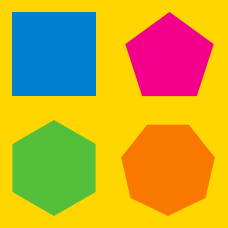Geometry

Regular Polygons - Properties of Regular Polygons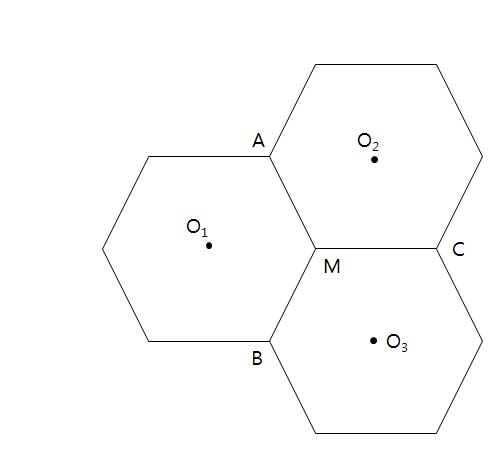The above diagram shows $3$ identical regular hexagons with side length $7.$ If $O_{1}, O_{2}$ and $O_{3}$ are their respective centers, what is the area of $\triangle O_{1}O_{2}O_{3}?$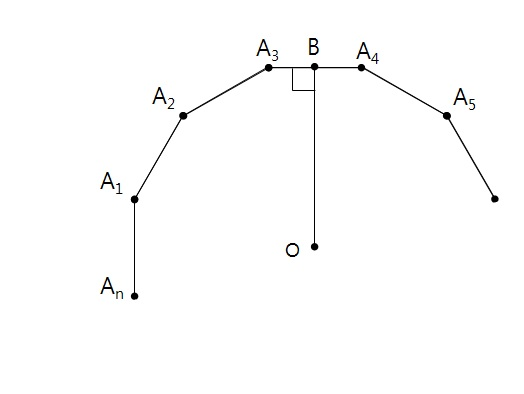The above diagram is part of a regular polygon with $n$ sides whose center of gravity is $O.$ If $n=10$ and the length of a side of the polygon is $30,$ what is the ratio of the areas of the inscribed circle and circumscribed circle?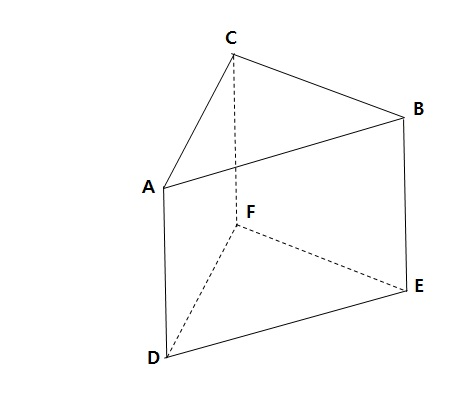The above diagram is a triangular prism whose faces are all regular polygons. If the length of $\overline{AB}$ is $3,$ what is the volume of the triangular prism?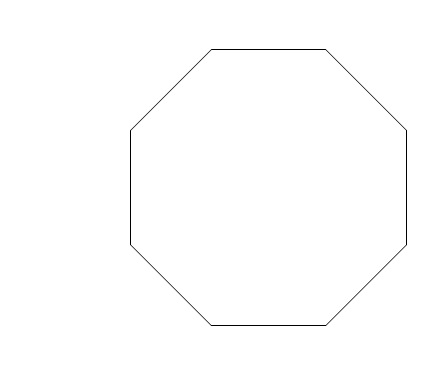The length of each side in the above regular octagon is $8.$ What is its area?

If each of the angles in a regular polygon is $120^{\circ}$, how many vertices does this polygon have?

×